Next: External Particles Up: QED Processes Previous: Higher-Order Calculations: Tree and

# Feynman Rules for Tree Graphs

We have now gained enough experience to extract a se of rules - the Feynman rules - which in princple will allow the calculation of any quantum electrodynamics processs no mater how complicated they are.

In the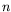'th order of perturbation theory one has to draw all possible topologically distinct Feynman diagrams withvertices that have the prescribed number of particles in the initial and final states (external lines).

The amplitudes of all graphs have to be added coherently, taking into account the following phase factors.

Remark to 1.

For the construction of Feynman graphs only the topological structure is important. Since the theory was formulated in a relativistically covariant way all possible time orderings are automatically taken into account. As long as ordering of the vertices along the fermions lines is kept, the graphs can be arbitrarily defomed without changing their meaning.

For the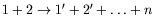process, the matrix element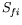can be written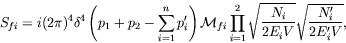(7.368)

where the essential physics is contained in the Lorentz covariant amplitude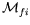.

The square root factors are due to the normalization of the incoming and outgoing plan waves. They are different for fermions and photons, this is expressed by the normalization factor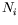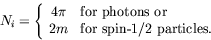(7.369)

The cross-section formula is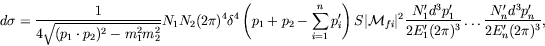(7.370)

where the degeneracy factor is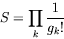(7.371)

if there are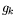particles of the kindin the final state.

The amplitudeis the invariant matrix element for the process under consideration, and is given by the Feynman rules of the theory. For particles with non-zero spin, unpolarized cross-sections are formed by averaging over initial spin components and summing over final.

SubsectionsNext: External Particles Up: QED Processes Previous: Higher-Order Calculations: Tree and
Douglas M. Gingrich (gingrich@ ualberta.ca)
2004-03-18